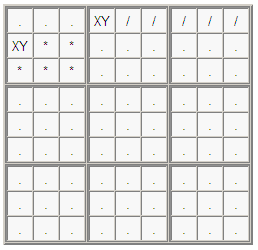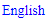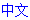# Almost Locked Candidates

The Almost Locked Candidates, or ALC, technique refers to a line-box intersection and a set of digits S such that:

• An Almost Locked Set (ALS) for the set of digits S exists in the line outside the intersection; and
• An ALS for the set of digits S exists in the box outside the intersection.

Then the following eliminations can be made:

• If all the cells in the line that are not in the intersection or the line-ALS contains no digits from S, then the digits from S can be eliminated from the cells in the box that are not in the intersection or the box-ALS.
• If all the cells in the box that are not in the intersection or the box-ALS contains no digits from S, then the digits from S can be eliminated from the cells in the line that are not in the intersection or the line-ALS.

### Illustration

The ALC technique is best illustrated by using a example.In the above grid, each cell marked XY means that it has the candidates X and Y, and each cell marked / means that it does not have X or Y as candidates. Now, if r1c4=X, then all the candidates for Y in row 1 are being confined in box 1. This triggers a Locked Candidates move, resulting in Y being eliminated from all cells in box 1 that are outside the intersection. But this also means that r2c1=X, so all the cells marked * cannot contain the digits X or Y. A similar deduction can be made if r1c4=Y. Therefore, in both cases, we can safely eliminate X and Y from all cells marked *.

### More examplesX, Y and Z can be eliminated from all cells marked *.

## Sudoku strategies

﻿## Printable Sudoku